Understanding Equation Engine

Oracle Corporation provides the PeopleSoft Equation Engine as a means for you to specify rules or equations as part of your business process. Equation Engine works best when you can specify an IF-THEN-ELSE validation resulting from a single call to an equation. For example, you may want to specify selection criteria by emplID or obtain a single number, string, or Boolean operator for that emplID.

The new version of the Equation Engine extends the capabilities of the original Equation Processor by adding looping and other constructs. It now can read any table for which you have security access and can perform various arithmetic operations and external call subroutines. In addition, security was added to ensure that control can be allocated based on your need and job function, which determine whether you need the ability to add, use, view, and modify equations, tables, and external routines.

With release 9, we added new syntax to enable you to perform mass actions—such as inserting, deleting, and updating rows in database tables—against the database using a single statement.

This section discusses:

• Online usage

• Architecture

• Language constructs

• Keyword syntax

• Basic language syntax

You use the Equation Editor page to enter information about an equation. You can compile, test, view in XML format, and print equations; the system displays the compile status of the equation. You can also create, edit, and delete the contents of equations. The equation is divided into three main sections: Equation Keyword, Operand Type, and Operand. You use various combinations of these main sections to define and create equations.

Access the Equation Editor page (select Set Up SACR, then select Common Definitions, then select Equation Engine, then select Equation Editor).

Image: Equation Editor page, FAPDPELLELIG example

This example illustrates the fields and controls on the Equation Editor page, FAPDPELLELIG example. You can find definitions for the fields and controls later on this page.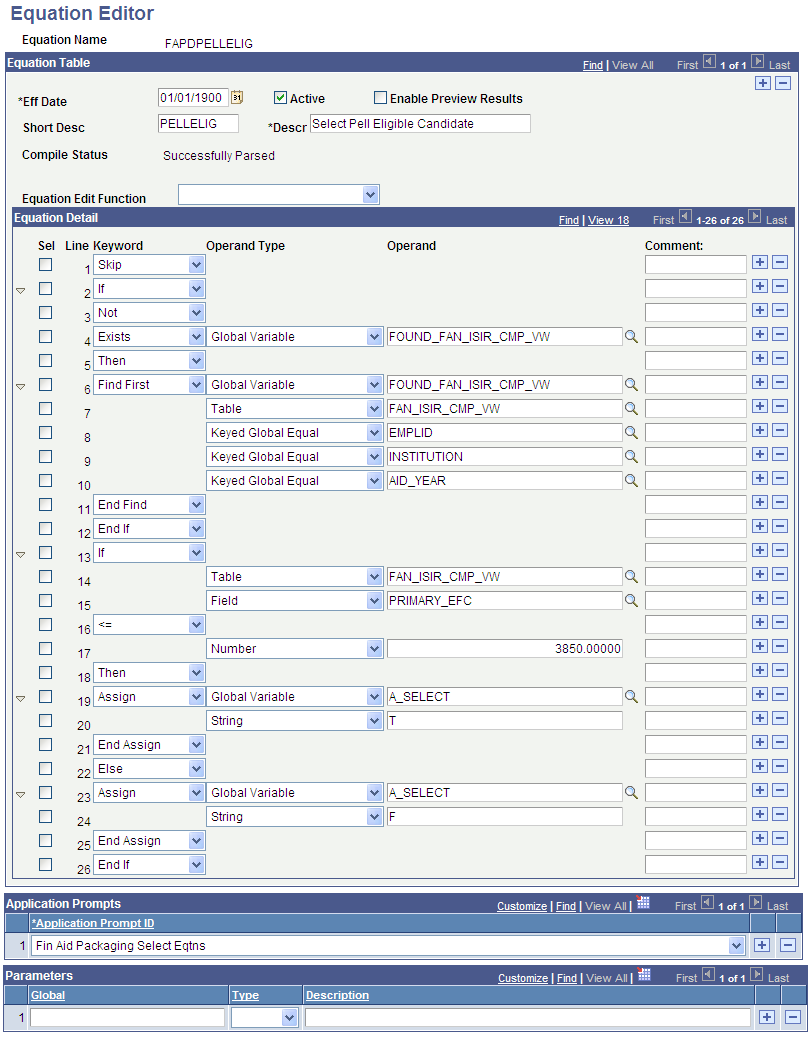Use the Sel (select) check boxes to select the lines to be affected by the Equation Edit function. Use the Comment fields to enter comments about an equation lines. Comments are information only.

To edit an equation, after you select a Sel check box, select one of the following options from the Equation Edit Function drop-down list box:

• Collapse Statements

• Compile

• Copy and Append to Clipboard

• Copy to Clipboard

• Cut and Append to Clipboard

• Cut to Clipboard

• Deselect All

• Expand Statements

• Paste After row

• Paste Before row

• Print

• Select All

• Select all between checks

• Test

• View in XML Format

Example of the Select All Between Checks Function

On the Equation Editor page, select the Sel check boxes for lines 2 and 12.

From the Equation Edit Function drop-down list box, select Select All Between Checks. When you click the Select All Between Checks option, the following page displays the selected check boxes for lines 2 through 12.

Image: Example for the Select All Between Checks option

This example illustrates the fields and controls on the Example for the Select All Between Checks option. You can find definitions for the fields and controls later on this page.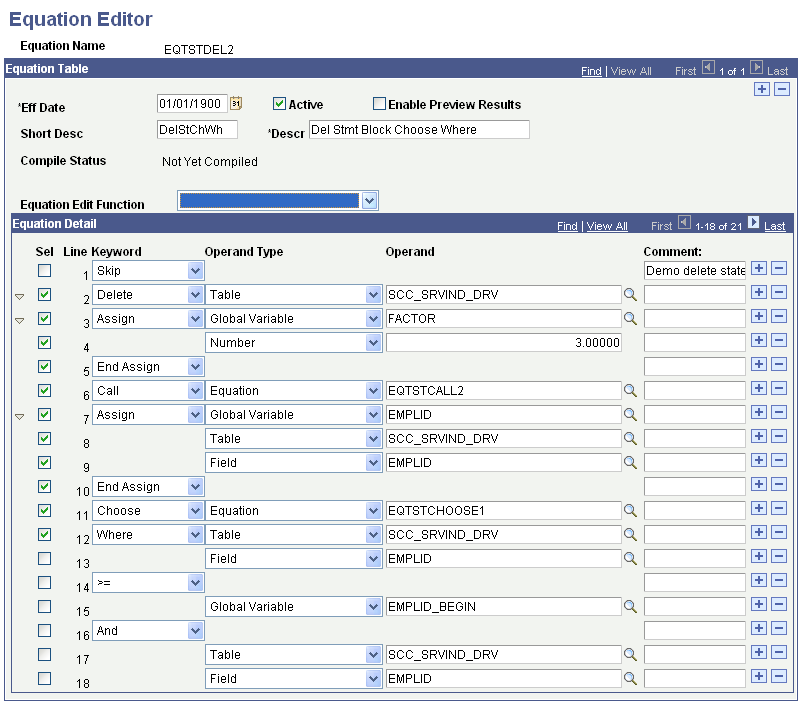Example of the Cut to Clipboard Function

From the Equation Edit Function drop-down list box, select Cut to Clipboard to copy the contents to the clipboard for later use. The selected lines are removed, as shown in the following example.

Image: Example for the Cut to Clipboard option

This example illustrates the fields and controls on the Example for the Cut to Clipboard option. You can find definitions for the fields and controls later on this page.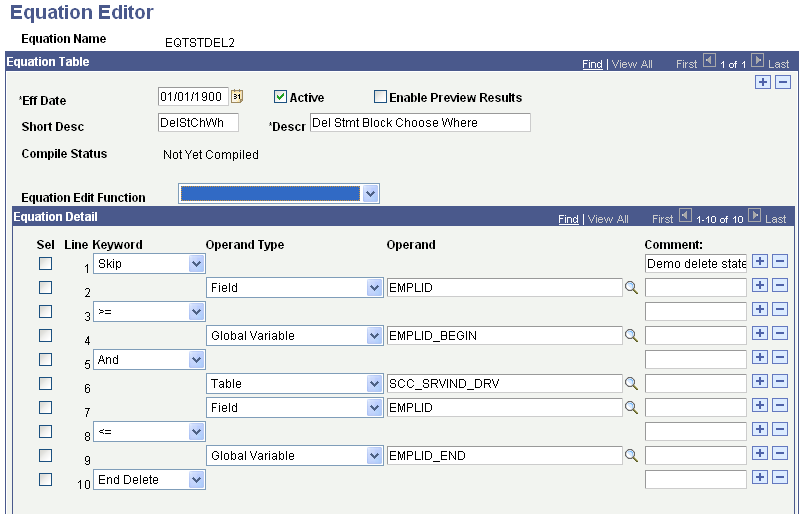Example of the Paste After Row Function

Select line 1 from the Sel check boxes.

From the Equation Edit Function drop-down list box, select Paste After row. Lines 2 through 12 are added from the clipboard, as shown in the following example:

Image: Example for the Paste After Row option

This example illustrates the fields and controls on the Example for the Paste After Row option. You can find definitions for the fields and controls later on this page.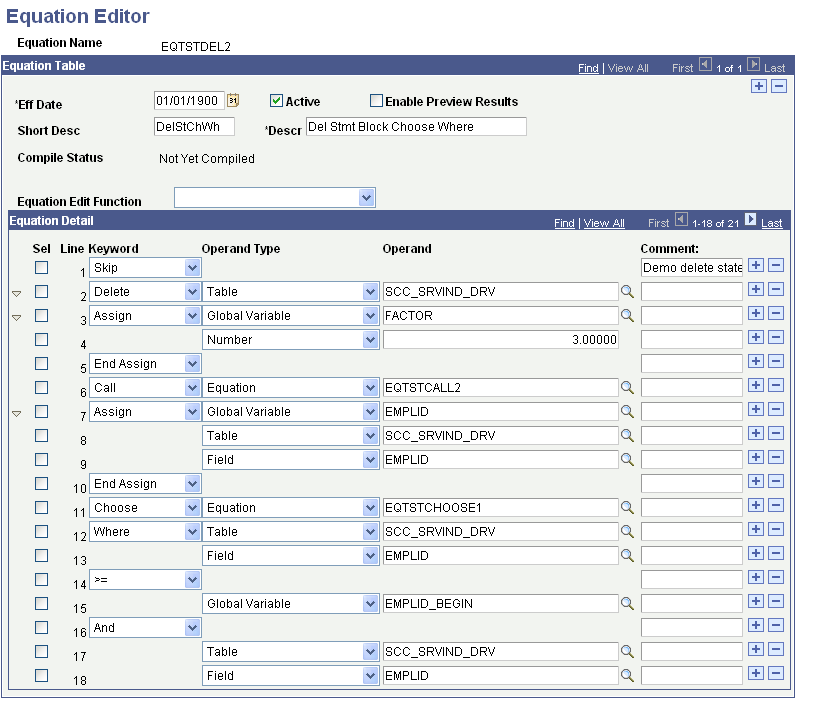Compile Function

From the Equation Edit Function drop-down list box, select Compile to check the syntax of the equation and to generate pseudo-code for the equation. You do not need to compile an equation; because the system compiles it for you when you run the equation. if you choose to compile an equation, you should compile the equation immediately after modifying it to check for syntax errors.

Example of Using the Expand and Collapse Buttons

The Equation Edit Function drop-down list box contains collapse and expand functions. To use them, you must first select the lines to be collapsed or expanded; then, you perform the function. For example, when you perform the Select All edit function followed by the Collapse Statements edit function, all collapsible statements in the equation are collapsed.

This is an example of the equation after all statements are collapsed:

Image: Example for the Collapse Statements option

This example illustrates the fields and controls on the Example for the Collapse Statements option. You can find definitions for the fields and controls later on this page.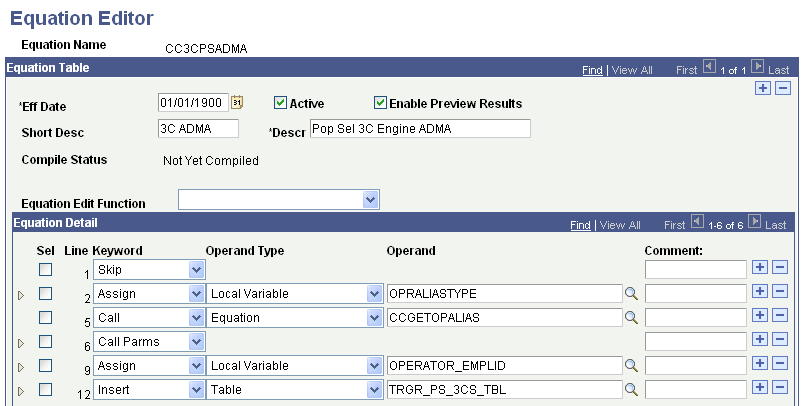Example of View In XML Format

When you select the View In XML Format edit function, the equation appears as an XML document in your browser. Because no style is associated with the XML document, the equation is presented as a document tree, in which the statements are indented and collapsible. The equation cannot be edited from the XML view of the equation.

Image: Example EQTSTDEL2 in XML format

This example illustrates the fields and controls on the Example EQTSTDEL2 in XML format. You can find definitions for the fields and controls later on this page.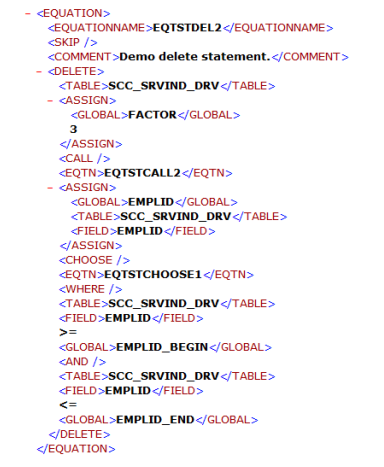Testing Equations

To test the equation, you may need to set up test data. In the previous example, the function of the equation was to add a few numbers together. No test data was needed because no global variables were needed. However, to test an equation that references keys in the table, passes global variables, or both you must provide the equation with test data.

To define and enter test data, go to the Equation Test Data page and define the name of the variable, its type, and its operand.

Access the Equation Test Data page (select Set Up SACR, then select Common Definitions, then select Equation Engine, then select Equation Test Data).

Image: Equation Test Data page

This example illustrates the fields and controls on the Equation Test Data page. You can find definitions for the fields and controls later on this page.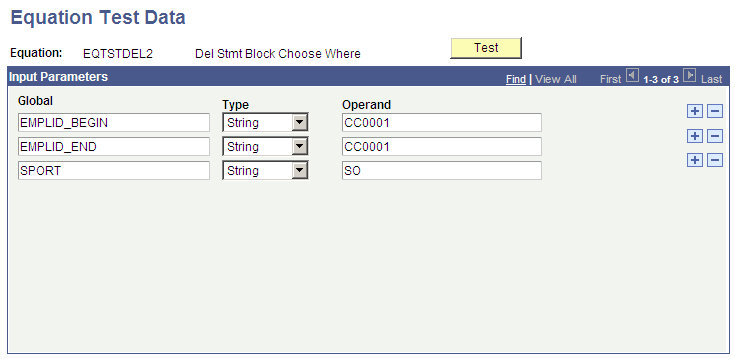After you define the necessary variables and enter data on the Equation Test Data page, click the Test button.

Note: You can also run a test from the Equation Editor by selecting Test from the Equation Edit Function drop-down list box.

When you click the Test button, the system displays the Equation Test Results, Process Messages tab:

Image: Equation Test Results page, Process Message tab

This example illustrates the fields and controls on the Equation Test Results page, Process Message tab. You can find definitions for the fields and controls later on this page.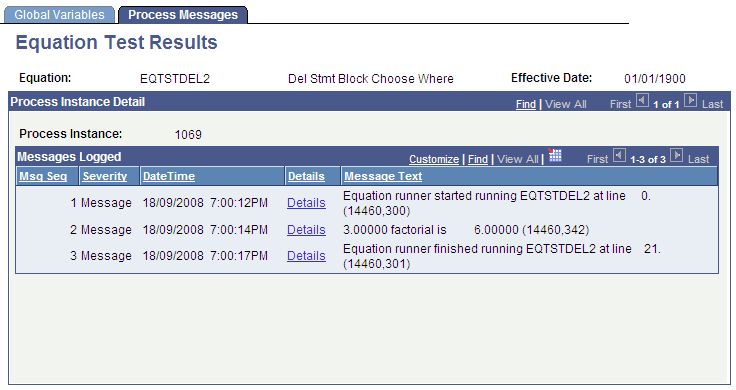This tab displays the messages associated with the equation test run.

The Equation Test Results, Global Variables tab displays the global variables used within the current equation run:

Image: Equation Test Results page, Global Variables tab

This example illustrates the fields and controls on the Equation Test Results page, Global Variables tab. You can find definitions for the fields and controls later on this page.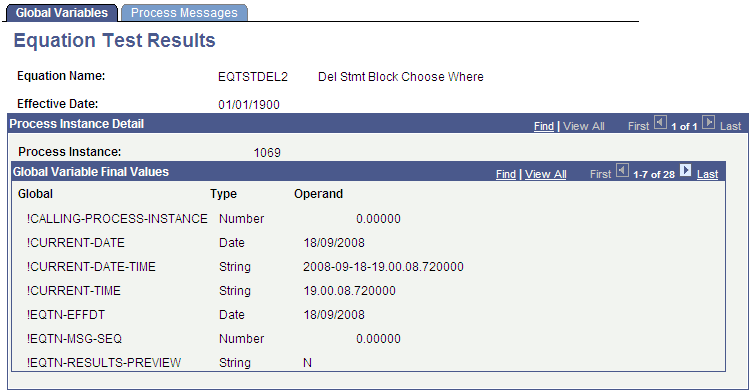The Print option in the Equation Edit function on the Equation Editor page enables you to print equations in a Crystal report format. When you select the Print option, the Equation Print page displays where you are required to enter a run control ID:

Image: Equation Print page

This example illustrates the fields and controls on the Equation Print page. You can find definitions for the fields and controls later on this page.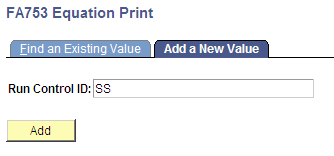.

Running the subsequent process yields a report that looks like this:

Image: EQTSTDEL2 Report

This example illustrates the fields and controls on the EQTSTDEL2 Report. You can find definitions for the fields and controls later on this page.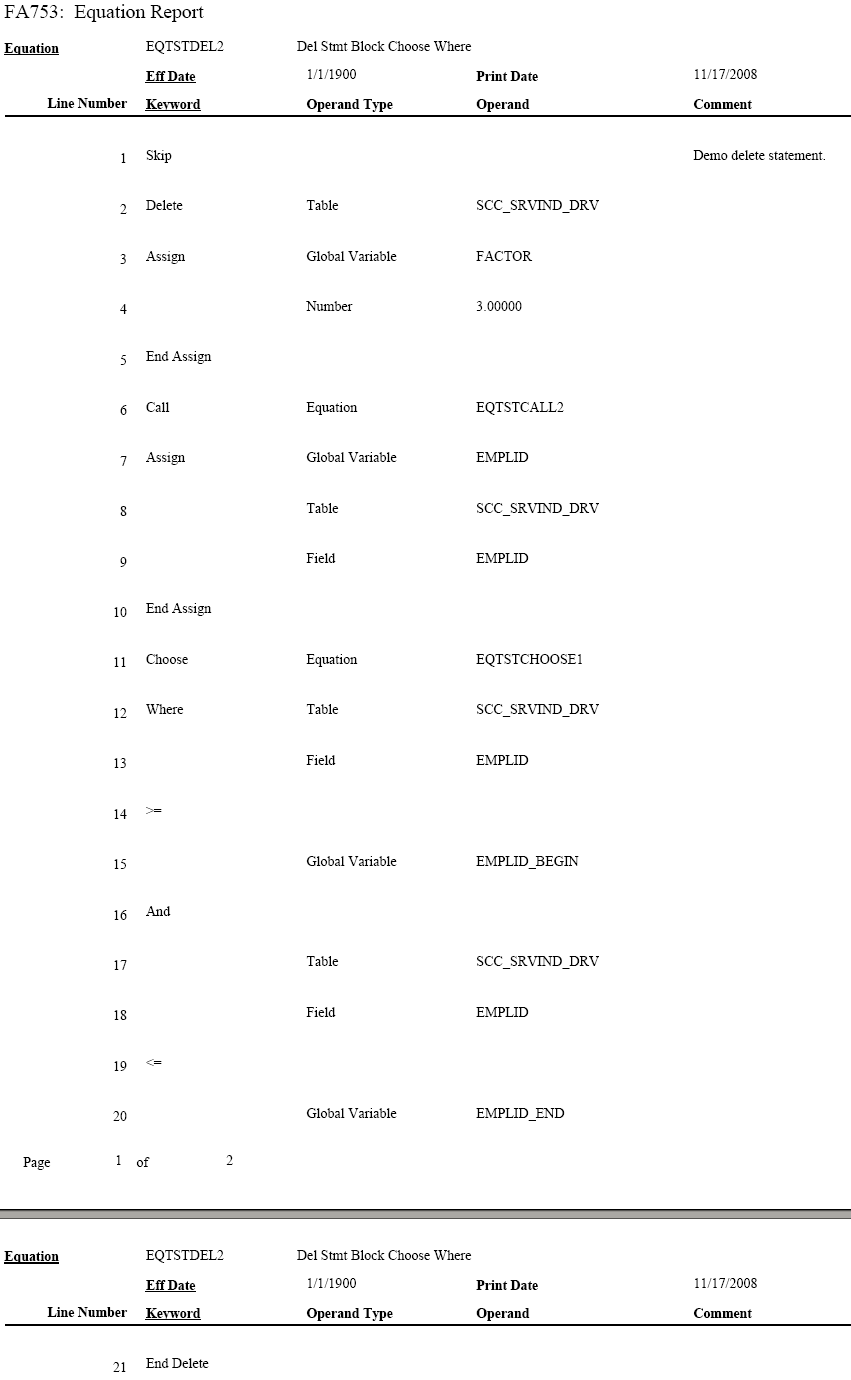The architectural changes in the current version of the Equation Engine are divided into two components: the Compiler and the Interpreter. The Compiler transforms the equation into pseudo-code (a pcode different from PeopleTools pcode), that is a type of platform-independent, low-level assembly code. The Interpreter reads the pcode and executes the equation directly. This method enables you to validate the syntax without running the equation. It also simplifies the logic of the programs.

Technical Notes

The architectural approach is a left-to-right look-ahead parser. Context sensitivity was pushed down to the lexical analyzer to make the parsing algorithm simpler.

Standard compiler design uses the driving routine as the parser. The parser makes calls to the lexical analyzer to retrieve units of logical language, called Tokens. It builds a parse tree, which is an internal representation of the structure of the program. It then reduces the parsing stack using the parse tree. For example, an if-then-else-end-if construct would be reduced to an if statement. Some of the reductions trigger calls to the object code generator, which generates the target language to be emitted. In this instance, the compiler output is the pcode. The design of the interpreter is simplified because the pcode is low-level.

Statement constructs were designed to end with END-XXXX phrases so that the parsing table state transitions are from top to bottom. When the equation is compiled by means of the compile equation edit function, additional up-front syntax checks are performed to provide more compile messages with more clarity about what is syntactically wrong with the equation. Additionally, if one of the more complicated statements has a syntax error, then a message appears showing the language syntax for that statement.

Equation Editor uses the language constructs discussed in the following topics.

Keywords

Keyword usage is mostly self-explanatory. Complex keywords will be explained later in the context of their syntactical constructs. These are the keywords:

• (

• )

• *

• +

• -

• /

• <

• <=

• <>

• =

• >

• >=

• And

• Assign

• Call

• Call Parms

• Choose

• Delete

• Else

• Else If

• End Assign

• End Call Parms

• End Delete

• End Find

• End If

• End Insert

• End Loop

• End Message

• End Restore

• End Save

• End Update

• Exists

• Exit Loop

• Find First

• Find Next

• From

• Halt

• If

• Insert

• Into

• Loop

• Message

• Not

• Or

• Restore

• Return

• Save

• Set

• Skip

• Then

• Trace

• Update

• Where

Global and Local Variables

Two types of variables are available in the Equation Engine: global and local. Data is passed to the Equation Engine by means of the global variable array or a process instance of the global variable tables that is defined by the Equation Test Data page or a calling program. Global variables are visible and can be referenced by any equation running or called. Local variables are visible only to the currently running equation.

Local and global variables can have a stem qualification. Stems are similar to arrays except that they have more versatility and are unbounded. You can assign a variable a name that ends with a period (.) to a default value (for example, DOGOWNER. = "unknown"), and then reference a subscript of that stem. If the subscript was not initialized with a value, then it will pick up the default value (for example, DOGOWNER.X has the value "unknown").

Stems are more versatile than arrays because the bounds of an array must be declared, but stems have no bounds to be declared and the subscripts do not have to be numeric. Therefore, you can have a subscript value of "Fido" instead of 7 (for example, Assign X = "Fido" and then Assign DOGOWNER.X = "Joe"). This construct not only allows a pseudo array processing capability, it also allows a pseudo pointer capability (for example, NODE.LEFT and NODE.RIGHT).

Read-only (system) variable names always begin with an exclamation mark.

Depending on which application is invoking the equation, different global variables may be assumed to be passed into the equation and different global variables (or none) are expected to be returned from the equation.

Global Variables Always Passed in for All Applications

The following global variables are always passed in:

• !CURRENT-DATE

• !CURRENT-DATE-TIME

• !CURRENT-TIME

• !EQUATION-NAME

• !PROCESS-INSTANCE

• !RUN-CNTL-ID

• OPRID (always read-only, FIND tests are forced to equality)

• PROCESS-INSTANCE (passed in)

• PROCESS_INSTANCE (actually used)

• RUN_CNTL_ID

Global Variables Passed in for the Forms Engine Financial Aid Award Notification Letter (FEFANLTR)

The following global variables are passed in:

FANLTR_SEQ

These global variables enable the equation to read the table RUNCNTL_FAN_SEQ, which provides these fields:

• INSTITUTION

• AID_YEAR

• PKG_AWARD_PERIOD

The equation can then assign global or local variables to these fields in order to loop through reading the table FAN_AWD_SEL_VW to determine whether or not to select an EMPLID. If the EMPLID is to be selected, the equation calls the SQL FANLTR_SELECT_STUDENT, passing in the EMPLID.

Note: No global variables are returned for FEFANLTR.

Global Variables Passed in for Financial Aid Packaging

The following global variables are passed in:

• EMPLID

• INSTITUTION

• AID_YEAR

• AWARD_PERIOD

• I_TRGT_FED_RMNEED

• I_TRGT_INST_RMNEED

• I_TRUE_FED_RMNEED

• I_INST_RMNEED

• I_LAST_AWD_ATM

• I_TOTAL_AWD_AMT

• WORK_FIELD_CHAR_01 through 05 (These global variables retain their values between equation calls. Initially, they are blank.)

• WORK_FIELD_NUM_01 through 05 (These global variables retain their values between equation calls. Initially, they are zero.)

• A_SELECT (initially blank)

• A_AMOUNT (initially zero)

The following global variables are examined upon return from the equation:

• A_SELECT (either "T" or "F", where "T" causes the student to be selected only for equations with the application prompt type of "Fin Aid Packaging Select Eqtns")

• A_AMOUNT (numeric dollar amount to be returned only for equations with the application prompt type of "Fin Aid Packaging Amount Eqtns")

Global Variables Passed in for Student Financials Tuition Calculation

The following global variables are passed in:

• EMPLID

• INSTITUTION

• STRM

• BILLING_CAREER

• STDNT_CAR_NBR

• A_SELECT (initially blank)

• A_AMOUNT (initially zero)

These global variables are examined upon return from the equation:

• A_SELECT (either "Y" or "N"; only the first character of the string is examined, but only for equations with the application prompt type of "Stdnt Fin Tuition Select Eqtns")

• A_AMOUNT (dollar amount to be returned, but only for equations with the application prompt type of "Stdnt Fin Tuition Amount Eqtns")

Global Variable Passed in for CommonLine and Common Record CommonLine Loan Validation

The global variable LN_ACTION_STATUS (initially "O" for OK) is passed in.

The following records are already fetched, and you can reference their fields:

• LN_EDIT_RUN_VW (for CL4 only)

• SFA_CRCEDRUN_VW (for CRC only)

• LOAN_ORIGNATN

• LN_TYPE_TBL

• LN_CL_PNOTE_VW (for CL4 only)

• SFA_CRC_PNOTE (for CRC only)

• LN_DEST_CATG_VW (for CL4 only)

• SFA_CRC_DCAT_VW (for CRC only)

The edit equation should set this global variable:

LN_ACTION_STATUS (looks for "O" for OK, or for "F" for Failed to pass the edit)

Every edit calls FA_EDGETDEST (CL4) or FACEDGETDEST (CRC), which sets the global DEST_ACTV. Next, the equation must test if the value is "Y" and if not, then return; otherwise, if the edit finds an error, it calls equation FA_EDLOGERR (CL4) or FACEDLOGERR (CRC), passing it the EQUATION_NAME local variable.

Global Variables Passed in for CommonLine and Common Record CommonLine Loan Hold/Release Processing

The following global variables are passed in:

• AID_YEAR

• EMPLID

• INSTITUTION

• STRM

• TABLE_ID (always "1")

• LOAN_TYPE

• LN_APPL_SEQ

• DISBURSEMENT_ID

• ITEM_TYPE

• RESULT (initially blank)

• HR_MSG_SET_NBR

• HR_MSG_NBR

These global variables are examined upon return from the equation:

• RESULT (either "PASS" or "FAILED")

• HR_MSG_SET_NBR and HR_MSG_NBR (string numeric Hold/Release message set number and message number are examined only if RESULT is "FAILED")

Global Variable Passed in for Population Selection

This global variable is passed in:

!CALLING-PROCESS-INSTANCE

No global variables are expected upon return; however, rows may be inserted into the target table.

Note: If a local or global variable does not have an assigned value when it is referenced, then the Equation Engine gives a runtime error. See the EXISTS keyword for more details.

Tables and Fields

You can read and use the table and fields by defining the tables to be used in the Equation Data Tables page.

Access the Equation Data Tables page (select Set Up SACR, then select Common Definitions, then select Equation Engine, then select Equation Data Tables).

Image: Equation Data Tables page

This example illustrates the fields and controls on the Equation Data Tables page . You can find definitions for the fields and controls later on this page.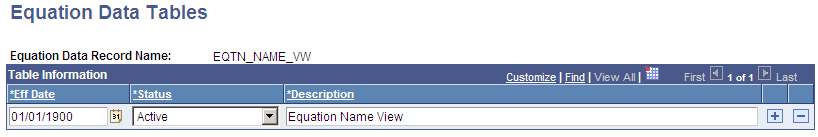If you reference a table-field but that table has not been read, then an implicit read will be performed with the assumption that the global variables are defined the same as the key to that table (for example, EMPLID, INSTITUTION, STRM, and so on). After the table is read, the first value of the read will be placed in that field.

Runtime Errors

If a variable is referenced but it has not been assigned a value, then a runtime error will occur. Also, if a table field is referenced and the fetch count is zero (no rows found), then a runtime error will also occur. One way of avoiding this error is to use the EXISTS keyword to determine whether the field or variable had a value and to either set a default value or issue a modified message and halt. This situation might occur if you call another equation that expects a local variable to be passed.

The maximum number of lines in an equation is 10,000.

Note: A truth value has an arithmetic value of zero for FALSE, and any other value is TRUE.

Logical Operators

Logical operators AND, OR, and NOT are used within an IF statement.

Syntax

<Condition1> <Logical Operator> <Condition2>

NOT <Condition>

Relational Operators

Relational operators: <, <=, =, >=, >, <>

Relational operators compare two expressions and yield a truth value. Use parentheses to group expressions and to enhance readability.

Syntax

<Expression1> <Relational Operator> <Expression2>

Arithmetic Operators

Arithmetic operators: +, -, *, /

Arithmetic operators apply to two expressions and yield an arithmetic result. Use parentheses to group expressions and to enhance readability.

Syntax

<Expression1> <Arithmetic Operator> <Expression2>

If rounding or truncation to an integer is necessary, then a call must be made to an external subroutine or equation (for example, the "ROUND" equation) that handles the task.

ASSIGN

Keywords: ASSIGN and END ASSIGN

The ASSIGN statement enables you to assign a value to either a local or a global variable. Every ASSIGN statement must be concluded with an END ASSIGN keyword.

Syntax

ASSIGN <Local or Global> <Variable Name> <Expression> END ASSIGN

Note: Local variables exist and can be referenced only within the context of the current equation, but global variables exist for the duration of the Equation Engine run. A global variable set in one equation can be referenced within a called equation and vice versa.

CALL

Keywords: CALL, CALL PARMS, and END CALL PARMS

The CALL statement enables an equation to call another equation, a callable SQL, or an external subroutine.

Syntax

This table lists examples of syntax for the keywords CALL, CALL PARMS, and END CALL PARMS:

Equation Keyword

Operand Type

Operand

CALL

<routine type>

<routine name>

CALL PARMS

LOCAL

<variable name>}...

END CALL PARMS

The CALL PARMS statement applies only to calling other equations or calling SQL. The types of routines are defined as equations, external subroutines, and SQL. You do not pass call parameters to external subroutines. To pass parameters to and from external subroutines, you must use global variables.

To use the Application Engine, you must call the Equation Engine from the Application Engine because the COBOL call to Application Engine is not supported.

For equation calls, the list of local variable names in the parameter list and their values are copied as separate local variables into the called equation. Local variables changed within the called equation are not changed upon the return to the calling equation. To pass a value back to a calling equation, the value must be returned by means of a global variable. Any returned global variable name should begin with the called equation name. If only one value is returned, the name should be <equation name>_RESULT. If you are returning a set of values, you can set the values into a global stem to encapsulate the result.

Equations can call themselves recursively; however, the maximum depth of equation call nesting is set at 1,000 calls. If your design requirements exceed the set amount, you must use a loop.

For SQL calls, the SQLID must exist in the Equation SQL setup table.

Access the Equation Callable SQL page (select Set Up SACR, then select Common Definitions, then select Equation Engine, then select Equation SQL Routines).).

The security for SQL calls is controlled through the operator alias type of EQS.

Note: You can use CALL SQL for updates, inserts, deletes, or small row retrieval selects (<1000), but use FIND statements instead of calling SQL with selects whenever possible.

CALL parameter order

The call parameter requires this order when calling a SQL:

Return code,

SQL row count,

Bind variable 1,

Bind variable 2,

.

Bind variable n,

Select variable 1,

Select variable 2,

.

Select variable n,

This is an example of a Call SQL Setup:

Image: Equation Callable SQL page

This example illustrates the fields and controls on the Equation Callable SQL page. You can find definitions for the fields and controls later on this page.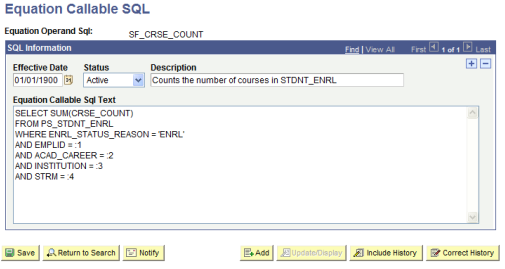All of the call parameters for SQL must be local variables. Global variables are not permitted. The select variables, if any, are updated by the call and are passed back to the calling equation, which differs from the effect of calling another equation and passing the local variables. If an equation modifies a passed local variable, that change is not reflected upon return to the calling program. However, when you call an SQL, the return code, SQL row count, and all of the select variables are modified by the call upon return from that called SQL.

Select variables are mapped to call parameters after the bind variables are mapped. The select variables must be stems to handle multiple rows of output (for example, EMPLID.1, EMPLID.2, and so on). Remember that a stem variable ends with a period and behaves similarly to an array.

DELETE

Keywords: DELETE, CHOOSE, WHERE, and END DELETE

Syntax

The following table shows examples of syntax for the keywords DELETE, CHOOSE, WHERE, and END DELETE:

Equation Keyword

Operand Type

Operand

DELETE

TABLE

<table name>

<statement block>

[CHOOSE

EQUATION

<equation name>]

WHERE

<where expression>

END DELETE

This statement deletes rows from the specified table, limited to the <where expression> evaluating to true (if it is supplied), and also limited to the choose equation (if it is supplied) returning a true value for each row.

<table name> is the name of a table to which the user has Equation Engine (EE) write authority.

<statement block> is any set of equation statements, except not DELETE, INSERT, nor UPDATE. Typically, if any statements exist , they are ASSIGN statements setting global variables that will be used within the CHOOSE equation, if it is specified.

<where expression> is passed to the database when you select which rows to delete. The database returns the resultant rowset to the delete statement. If no where clause is specified, then all rows in the table are processed. The where clause may mention table-fields from the table operand of the delete keyword as well as any other table to which the user has EE read authority. The other tables, if mentioned, do not get rows delete, but rather may be used to limit which rows will be deleted by joining to the table in which rows are being deleted.

CHOOSE clause is optional and if specified will cause EE to call the choose equation once for each row in the rowset to be processed. Typically, any necessary global variables needed by the choose equation to do its function were set within the aforementioned <statement block>. After calling the choose equation, the choose clause will first look to see if there is a global variable with the same name as the choose equation name. If there is, then it will inspect it for a value of the number zero, which it will interpret as a false condition. Any other value is a true condition. If a true condition is returned, then the row is selected for processing. If it does not find the global variable with the same name as the choose equation name, then it will look for the global variable named A_SELECT. If a value is set for A_SELECT, it can have one the following values, which will indicate a true condition: T, TRUE, t, true, Y, YES, y, or yes. Any other value is considered to be a false condition.

EXISTS

Keyword: EXISTS

The EXISTS statement tests to determine whether a variable exists or whether any rows were returned when the EXISTS statement is applied against a table. It cannot be used to validate field names.

Syntax

This table shows example syntax for the keyword EXISTS:

Equation Keyword

Operand Type

Operand

EXISTS

<object type>

<object name>

<object type> is a local variable, global variable, or table name.

It returns a truth value and is typically used within an IF statement. It is commonly used to validate variables or tables prior to its usage to avoid runtime errors. If the variable has not been initialized, then the calling program must set default values to variables.

Example:

If you establish an equation (COMPUTE_ANGLE) that will be called by other equations, it requires the following parameters to be passed:

• XROW

• YROW

• DISTANCE

The COMPUTE_ANGLE tests to make sure XROW, YROW, and DISTANCE exist. If they do not, then variables can be set to default values or you can return a message to the calling equation.

When referencing table fields, the EXISTS statement determines whether a row has been selected for a given table name using either an implicit or explicit FIND statement.

FIND

Keywords: FIND FIRST, FIND NEXT, and END FIND

The FIND FIRST keyword positions the equation in the first row of the specified table for the given key values and key relational operators. The FIND NEXT keyword moves to the next row within that previously specified set of key values and key relational operators. If you need to process multiple rows of data within a single call to an equation, you should use a FIND FIRST followed by a LOOP statement that contains a FIND NEXT near the end of the statement. Test the find success variable to determine when to exit the loop.

Note: An implicit FIND is performed when a field of a table is referenced and no FIND FIRST was previously issued. In the implicit FIND, all relational operators are considered equal. The key values are obtained from the global variables defined within the equation and from where the global variable names match the key field names of the table.

Syntax

This table shows examples of syntax for the keywords FIND FIRST, END FIND, and FIND NEXT:

Equation Keyword

Operand Type

Operand

FIND FIRST

<local or global>

<find success variable>

TABLE

<table name>

{<find condition>

<field name>}

END FIND

N/A

N/A

N/A

FIND NEXT

<local or global>

<find success variable>

TABLE

<table name>

END FIND

The find success variable can be a local or global variable containing a truth value that indicates whether a row was found.

The find condition operators (EQUAL, GREATER-THAN, and GREATER-THAN-OR-EQUAL-TO) compare the assign variable against the table. The variables must have the same names as the fields of the table.

Every FIND statement must end with an END FIND keyword.

Example of Find Setup

This table shows example syntax for the keyword FIND FIRST:

Equation Keyword

Operand Type

Operand

FIND FIRST

LOCAL

FOUND_STUDENT

TABLE

STDNT_FA_TERM

KEYED LOCAL EQUAL

EMPLID

KEYED LOCAL EQUAL

INSTITUTION

KEYED LOCAL EQUAL

STRM

END FIND

An alternative to looping through successive FIND NEXT statements is to issue a single CALL SQL statement, where the called SQL is a select statement. The values returned are placed into local stem variables, of which there is an upper limit of one thousand. If you exceed the limit, the equation engine will halt with a runtime error. For a large number of returned rows, it is best to process them with successive FIND NEXT statements and handle the data one row at a time. If you need to do changes en masse, you can call SQL to update temporary tables and avoid row-by- row processing, this technique is preferred.

HALT

Keyword: HALT

The HALT statement stops the execution run of all equations. The Equation Engine immediately returns control to its calling program; control is not returned to a calling equation. This keyword is typically used in the event of a catastrophic error. For example, a test is performed to determine whether a row exists for an emplID. If it does not, you issue a customized message to stop the equations.

IF

Keywords: IF, THEN, ELSE IF, ELSE, and END IF

The IF statement executes different statements depending on truth values within its conditions. Each IF statement block must conclude with an END IF keyword.

Syntax

IF

<condition-1>

THEN

<statement block-1>

[ELSE IF

<condition-n>

THEN

<statement block-n>] …

[ELSE

<statement block-n+1>]

END IF

Image: Equation Editor page, if and End If Example

This example illustrates the fields and controls on the Equation Editor page, if and End If Example. You can find definitions for the fields and controls later on this page.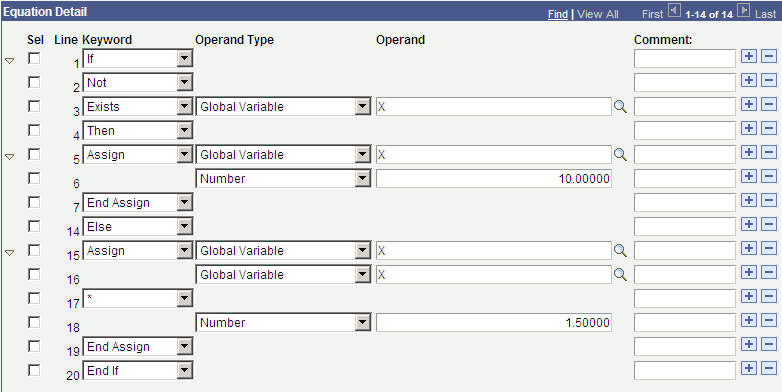The ELSE IF and ELSE expressions are optional. The ELSE IF expression enables you to create a case control structure so a single END IF can be used instead of nesting IF's. You can have zero, one, or several "ELSEIF … THEN …" constructs within a single IF statement.

Nested IF statements are allowed within the equation. A statement block can contain any other statements, including an IF statement.

INSERT

Keywords: INSERT, INTO, FROM, CHOOSE, WHERE, and END INSERT

Syntax

This table shows examples of syntax for the keywords INSERT, INTO, FROM CHOOSE, WHERE and END INSERT:

Equation Keyword

Operand Type

Operand

INSERT

TABLE

<table name>

[<statement block>]

<INTO

FIELD

<field name>

FROM

<expression>

>...

[CHOOSE

EQUATION

<equation name>]

WHERE

<where expression>

END INSERT

This statement inserts rows into the specified table, limited to the <where expression> evaluating to true (if it is supplied), and then also limited to the choose equation (if it is supplied) returning a true value for each row.

<table name> is the name of a table to which the user has Equation Engine (EE) write authority.

See the DELETE statement for details on the <statement block>, CHOOSE, and WHERE clauses.

INTO specifies a field that is to have a value when the row is inserted. Its value is set from the result of the <expression> in the FROM clause. If a field is marked as being 'required' within the Application Designer definition for that record, then that field must have an INTO clause associated with it. If the FROM <expression> specifies a global stem, in the format of <recname>.<fieldname>.<anyname>, then the <anyname> qualified is assumed to be an index into the global stem with the name <recname>.<fieldname>, and the ending limit of that index will be assumed to be the <recname>.!COUNT global value. (See alternate insert syntax.)

Alternate Syntax

This table shows examples of syntax for the keywords INSERT, CHOOSE, WHERE, and END DELETE:

Equation Keyword

Operand Type

Operand

INSERT

GLOBAL

<recname>.

[<statement block>]

[CHOOSE

EQUATION

<equation name>]

WHERE

<where expression>

END DELETE

This statement acts like the INSERT statement, except that the recname is followed by a dot, which makes the target of the insert a global stem. The value of <recname>.!COUNT will contain the number of rows in the global stem. If X is a global number holding a row number, then <recname>.<fieldname>.X will contain the value of the field <fieldname> in the table <recname> at row number X.

LOOP

Keywords: LOOP, EXIT LOOP, and END LOOP

The LOOP statement permits a block of statements to be run repetitively. Each LOOP statement block must end with an END LOOP statement.

Syntax

LOOP

<statement block>

END LOOP

Every loop block should include an EXIT LOOP keyword to stop the loop when the desired condition is reached. A RETURN or HALT statement can also be substituted, though it is discouraged because it makes your equations more difficult to maintain.

MESSAGE

Keywords: MESSAGE and END MESSAGE

The MESSAGE statement writes a message to the message log file (PS_MESSAGE_LOG) under the current process instance. It can contain up to 10 values, each of which can be a constant string, constant number, local or global variable, or table-field.

Syntax

This table shows an example of syntax for the keyword MESSAGE:

Equation Keyword

Operand Type

Operand

MESSAGE

{<data type>

<data value>}

END MESSAGE

<data type> : STRING, DATE, NUMBER, LOCAL, GLOBAL, or TABLE xxx FIELD yyy

Note: Writing a message triggers a commit to be performed.

Keyword: RESTORE

Syntax

This table shows example syntax for the keyword RESTORE:

Equation Keyword

Operand Type

Operand

RESTORE

STRING

<equation global space

[GLOBAL

<global variable name>]...

END RESTORE

The RESTORE statement restores the specified global variable names from the previously created equation global space that is specified. If no global variable names are listed, then all global variables are restored, excluding read-only system variables.

RETURN

Keyword: RETURN

The RETURN statement causes the equation currently executing to stop processing, and it returns control to the calling equation. If no calling equation exists, then control returns to the program that called the Equation Engine. HALT and RETURN act the same if the Equation Engine calls only one equation and that equation does not call any others. An implicit RETURN statement is appended to the end of every equation if one was not placed there explicitly. The RETURN statement has no parameters. If you want to pass a parameter back to a calling equation, you must use global variables.

Keyword: SAVE

Syntax

This table shows example syntax for the keyword SAVE:

Equation Keyword

Operand Type

Operand

SAVE

STRING

<equation global space

[GLOBAL

<global variable name>]...

END SAVE

The SAVE statement saves the specified global variable names to the specified equation global space. If no global variable names are listed, then all global variables are saved into the global space.

SKIP

Keyword: SKIP

The SKIP statements are used to make a program more readable. It allows you to break up the program statements, and it can simplify your IF logic.

Note: A SKIP statement does not affect your program.

TRACE

Keyword: TRACE

The TRACE statement causes the equation currently executing to start or stop displaying the type of trace information specified by the operand type.

Syntax

This table shows example syntax for the keyword TRACE:

Equation Keyword

Operand Type

Operand

TRACE

{<trace type>

<trace status>}...

END MESSAGE

<trace type>: SOURCE, PCODE or SQL.

<trace status>: ON or OFF

Tracing SOURCE causes the line numbers of the source equation to appear to the COBOL log file as they are run; intermediate values also appear. Tracing PCODE causes the pseudo-code functions, their parameters, and their line numbers to appear as they are run; intermediate values and function results also appear. Tracing SQL causes the actual SQL that is run to appear; bind variables and values, if any, as well as the return code and row count or dml count for the SQL also appear. Retrieved table and-field values do not appear. To enable more than one trace type at the same time, issue more that one trace statement. Tracing remains in effect globally until disabled. Therefore, a trace enabled in a called equation will continue tracing when control returns to the calling equation, and vice versa.

UPDATE

Keywords: UPDATE, SET, CHOOSE, WHERE, and END UPDATE

Syntax

The table shows example syntax for the keyword UPDATE:

Equation Keyword

Operand Type

Operand

UPDATE

TABLE

<table name>

[<statement block>]

<SET

FIELD

<field name>

<expression>

>...

[CHOOSE

EQUATION

<equation name>]

WHERE

<where expression>

END UPDATE

This statement updates rows in the specified table, limited to the <where expression> evaluating to true. See the DELETE statement for details on the <statement block>, CHOOSE, and WHERE clauses.

SET clause sets the specified <field name> in the <table name> to the value of the <expression>.

This table lists the parse objects used in the Equation Editor:

Parse Object

Elaboration

Code Gen

(Skip reduction if next token is . . .)

<PROGRAM>

<STMT BLOCK>

<ENDPROGRAM TOKEN>

ACCEPT

<STMT BLOCK>

<STMT BLOCK> <STMT>

<STMT BLOCK>

<STMT>

<STMT>

<IF STMT>

<STMT>

<LOOP STMT>

<STMT>

<ASSIGN STMT>

<STMT>

<CALL STMT>

<STMT>

<RETURN STMT>

<STMT>

<HALT STMT>

<STMT>

<MESSAGE STMT>

<STMT>

<EXIT LOOP STMT>

<STMT>

<SKIP STMT>

<STMT>

<FIND STMT>

<STMT>

<FIND NEXT STMT>

<STMT>

<TRACE STMT>

<STMT>

<DELETE STMT>

<STMT>

<INSERT STMT>

<STMT>

<RESTORE STMT>

<STMT>

<SAVE STMT>

<STMT>

<UPDATE STMT>

<IF STMT>

<IF START> <OR EXPR> <THEN PHRASE> <IF CLOSURE>

IFSTMT

<IF CLOSURE>

<ELSIF THEN LIST> <STMT BLOCK> <IF ENDING>

<IF CLOSURE>

<STMT BLOCK> <IF ENDING>

<IF ENDING>

<ELSE PHRASE> <STMT BLOCK> <ENDIF TOKEN>

<IF ENDING>

<ENDIF TOKEN>

<ELSIF THEN LIST>

<ELSIF THEN LIST> <ELSIF THEN PHRASE>

<ELSIF THEN LIST>

<ELSIF THEN PHRASE>

<ELSIF THEN PHRASE>

<STMT BLOCK> <ELSIF PHRASE> <OR EXPR> <THEN PHRASE>

<IF START>

<IF TOKEN>

IFSTART

<LOOP STMT>

<LOOP START> <STMT BLOCK> <ENDLOOP TOKEN>

LOOPEND

<LOOP START>

<LOOP TOKEN>

LOOPSTART

<ASSIGN STMT>

<ASSIGN TOKEN> <ASSIGNABLE DATATYPE> <OR EXPR> <ENDASSIGN TOKEN>

ASSIGN

<OR EXPR>

<OR EXPR> <OR TOKEN> <AND EXPR>

OR

<OR EXPR>

<AND EXPR>

<AND TOKEN>

<AND EXPR>

<AND EXPR> <AND TOKEN> <NOT EXPR>

AND

<AND EXPR>

<NOT EXPR>

<NOT EXPR>

<REL EXPR>

<EQUAL TOKEN> OR <LESS TOKEN> OR <LESS OR EQUAL TOKEN> OR <GREATER TOKEN> OR <GREATER OR EQUAL TOKEN> OR <UNEQUAL TOKEN>

<NOT EXPR>

<NOT TOKEN> <NOT EXPR>

NOT

<NOT EXPR>

<NOT TOKEN> <OR EXPR>

NOT

<REL EXPR>

<REL EXPR> <COMPARE LESS> <SUM EXPR>

LESS

<MINUS TOKEN> OR <PLUS TOKEN> OR <DIVIDE TOKEN> OR <MULTIPLY TOKEN>

<REL EXPR>

<REL EXPR> <COMPARE EQUAL> <SUM EXPR>

EQUAL

<MINUS TOKEN> OR <PLUS TOKEN> OR <DIVIDE TOKEN> OR <MULTIPLY TOKEN>

<REL EXPR>

<REL EXPR> <COMPARE LESS EQUAL> <SUM EXPR>

LESSEQUAL

<MINUS TOKEN> OR <PLUS TOKEN> OR <DIVIDE TOKEN> OR <MULTIPLY TOKEN>

<REL EXPR>

<REL EXPR> <COMPARE GREATER> <SUM EXPR>

GREATER

<MINUS TOKEN> OR <PLUS TOKEN> OR <DIVIDE TOKEN> OR <MULTIPLY TOKEN>

<REL EXPR>

<REL EXPR> <COMPARE GREATER EQUAL> <SUM EXPR>

GREATEREQUAL

<MINUS TOKEN> OR <PLUS TOKEN> OR <DIVIDE TOKEN> OR <MULTIPLY TOKEN>

<REL EXPR>

<REL EXPR> <COMPARE UNEQUAL> <SUM EXPR>

UNEQUAL

<MINUS TOKEN> OR <PLUS TOKEN> OR <DIVIDE TOKEN> OR <MULTIPLY TOKEN>

<REL EXPR>

<SUM EXPR>

<MINUS TOKEN> OR <PLUS TOKEN>

<SUM EXPR>

<SUM EXPR> <PLUS TOKEN> <PRODUCT EXPR>

<DIVIDE TOKEN> OR <MULTIPLY TOKEN>

<SUM EXPR>

<SUM EXPR> <MINUS TOKEN> <PRODUCT EXPR>

SUBTRACT

<DIVIDE TOKEN> OR <MULTIPLY TOKEN>

<SUM EXPR>

<PRODUCT EXPR>

<DIVIDE TOKEN> OR <MULTIPLY TOKEN>

<PRODUCT EXPR>

<PRODUCT EXPR> <MULTIPLY TOKEN> <MONADIC EXPR>

MULTIPLY

<PRODUCT EXPR>

<PRODUCT EXPR> <DIVIDE TOKEN> <MONADIC EXPR>

DIVIDE

<NEGATE TOKEN> <OR EXPR>

NEGATE

NEGATE

<PRODUCT EXPR>

<VALUE>

<LEFTPAREN TOKEN> <OR EXPR> <RIGHTPAREN TOKEN>

<INTO TOKEN> <STRING LITERAL TOKEN>

INTOSTRHDR

<VALUE>

<NUMERIC LITERAL TOKEN>

NUMBER

<VALUE>

<STRING LITERAL TOKEN>

STRING

- <VALUE>

<DATE LITERAL TOKEN>

DATE

<VALUE>

<DATA VALUE>

<VALUE>

<EXISTS STMT>

<DATA VALUE>

<TABLEFIELD>

GETTBLFLDVALUE

<DATA VALUE>

<GLOBALFIELD>

GETGLOBVALUE

<DATA VALUE>

<LOCALFIELD>

GETLOCVALUE

<FIND STMT>

<FIND TOKEN> <ASSIGN FIND DATATYPE> <TABLENAME> <KEY IDENTIFIER LIST> <ENDFIND TOKEN>

FIND

<FIND NEXT STMT>

<FIND NEXT TOKEN> <ASSIGN FIND DATATYPE> <TABLENAME> <ENDFIND TOKEN>

FINDNEXT

<CALL STMT>

CALL

<CALLPARMS TOKEN>

<CALL STMT>

CALLWITHPARMS

<CALL TOKEN> <EQUATIONTYPE TOKEN> <IDENTIFIER TOKEN>

CALLEQUATION

<CALL TOKEN> <EXTERNALTYPE TOKEN> <IDENTIFIER TOKEN>

CALLEXTERNAL

<CALL TOKEN> <APPENGINETYPE TOKEN> <IDENTIFIER TOKEN>

CALLAPPENG

<CALL TOKEN> <SQLTYPE TOKEN> <IDENTIFIER TOKEN>

CALLSQL

<CALL PARMS STMT>

<CALLPARMS TOKEN> <CALL PARM LIST> <ENDCALLPARMS TOKEN>

<CALL PARM LIST>

<CALL PARM LIST> <CALL PARM>

<CALL PARM LIST>

<CALL PARM>

<CALL PARM>

<CALL LOCALFIELD>

<CALL LOCALFIELD>

<CALL LOCALTYPE TOKEN> <IDENTIFIER TOKEN>

CALLLOCAL

<RETURN STMT>

RETURN

<HALT STMT>

<HALT TOKEN>

HALT

<MESSAGE STMT>

<MESSAGE TOKEN> <MESSAGE PARM LIST> <ENDMESSAGE TOKEN>

MSG

- <MESSAGE PARM LIST>

<MESSAGE PARM LIST> <MESSAGE PARM>

<MESSAGE PARM LIST>

<MESSAGE PARM>

MSGPARM

<MESSAGE PARM>

<MSG NUMERIC LITERAL TOKEN>

MSGNUMBER

<MESSAGE PARM>

<MSG STRING LITERAL TOKEN>

MSGSTRING

<MESSAGE PARM>

<MSG DATE LITERAL TOKEN>

MSGDATE

<MESSAGE PARM>

<MSG DATA VALUE>

<MSG DATA VALUE>

<MSG TABLEFIELD>

<MSG DATA VALUE>

<MSG GLOBALFIELD>

<MSG DATA VALUE>

<MSG LOCALFIELD>

<MSG TABLENAME>

<MSG TABLETYPE TOKEN> <IDENTIFIER TOKEN>

MSGTABLENAME

<INSERT START>

<INSERT HDR> <GLOBALTYPE TOKEN> <IDENTIFIER TOKEN>

INSGLOB

<MSG TABLEFIELD>

<MSG TABLENAME> <FIELDTYPE TOKEN> <IDENTIFIER TOKEN>

MSGTABLEFIELD

<MSG GLOBALFIELD>

<MSG GLOBALTYPE TOKEN> <IDENTIFIER TOKEN>

MSGGLOBAL

<MSG LOCALFIELD>

<MSG LOCALTYPE TOKEN> <IDENTIFIER TOKEN>

MSGLOCAL

<EXIT LOOP STMT>

<EXITLOOP TOKEN>

EXITLOOP

<EXISTS STMT>

<EXISTS TOKEN> <EXISTS GLOBALTYPE TOKEN> <IDENTIFIER TOKEN>

EXISTSGLOBAL

<EXISTS STMT>

<EXISTS TOKEN> <EXISTS LOCALTYPE TOKEN> <IDENTIFIER TOKEN>

EXISTSLOCAL

<EXISTS STMT>

<EXISTS TOKEN> <EXISTS TABLETYPE TOKEN> <IDENTIFIER TOKEN>

EXISTSTABLE

<ASSIGNABLE DATATYPE>

<ASGN GLOBALFIELD>

<ASSIGNABLE DATATYPE>

<ASGN LOCALFIELD>

<ASSIGN FIND DATATYPE>

<FIND GLOBALFIELD>

<ASSIGN FIND DATATYPE>

<FIND LOCALFIELD>

<INSERT START>

<INSERT HDR> <TABLETYPE TOKEN> <IDENTIFIER TOKEN>

INSTABLE

<DELETE START>

<DELETE HDR> <TABLETYPE TOKEN> <IDENTIFIER TOKEN>

DELTABLE

<UPDATE START>

<UPDATE HDR> <TABLETYPE TOKEN> <IDENTIFIER TOKEN>

UPDTABLE

<TABLENAME>

<TABLETYPE TOKEN> <IDENTIFIER TOKEN>

TABLENAME

<TABLEFIELD>

<TABLENAME> <FIELDTYPE TOKEN> <IDENTIFIER TOKEN>

TABLEFIELD

<GLOBALFIELD>

<GLOBALTYPE TOKEN> <IDENTIFIER TOKEN>

GLOBALFIELD

<ASGN GLOBALFIELD>

<ASGN GLOBALTYPE TOKEN> <IDENTIFIER TOKEN>

ASGNGLOBAL

<LOCALFIELD>

<LOCALTYPE TOKEN> <IDENTIFIER TOKEN>

LOCALFIELD

<FIND LOCALFIELD>

<FIND LOCALTYPE TOKEN> <IDENTIFIER TOKEN>

FINDLOCAL

<FIND GLOBALFIELD>

<FIND GLOBALTYPE TOKEN> <IDENTIFIER TOKEN>

FINDGLOBAL

<ASGN LOCALFIELD>

<ASGN LOCALTYPE TOKEN> <IDENTIFIER TOKEN>

ASGNLOCAL

<KEY IDENTIFIER LIST>

<KEY IDENTIFIER LIST> <KEY IDENTIFIER>

<KEY IDENTIFIER LIST>

<KEY IDENTIFIER>

<KEY IDENTIFIER>

<KEY LOCAL EQUAL TOKEN> <IDENTIFIER TOKEN>

KEYLOCEQ

<KEY IDENTIFIER>

<KEY LOCAL GREATER TOKEN> <IDENTIFIER TOKEN>

KEYLOCGT

<KEY IDENTIFIER>

<KEY LOCAL GREATER EQUAL TOKEN> <IDENTIFIER TOKEN>

KEYLOCGE

<KEY IDENTIFIER>

<KEY GLOBAL EQUAL TOKEN> <IDENTIFIER TOKEN>

KEYGLOBEQ

<KEY IDENTIFIER>

<KEY GLOBAL GREATER TOKEN> <IDENTIFIER TOKEN>

KEYGLOBGT

<KEY IDENTIFIER>

<KEY GLOBAL GREATER EQUAL TOKEN> <IDENTIFIER TOKEN>

KEYGLOBGE

<COMPARE LESS>

<LESS TOKEN>

<COMPARE LESS EQUAL>

<LESS OR EQUAL TOKEN>

<COMPARE EQUAL>

<EQUAL TOKEN>

<COMPARE GREATER>

<GREATER TOKEN>

<COMPARE GREATER EQUAL>

<GREATER OR EQUAL TOKEN>

<COMPARE UNEQUAL>

<UNEQUAL TOKEN>

<THEN PHRASE>

<THEN TOKEN>

THEN

<ELSE PHRASE>

<ELSE TOKEN>

ELSE

<ELSIF PHRASE>

<ELSIF TOKEN>

ELSIF

<SKIP STMT>

NONE

<TRACE STMT>

<TRACE TOKEN> <TRACE SQL TOKEN> <TRACE ON OR OFF>

TRACESQL

<TRACE STMT>

<TRACE TOKEN> <TRACE PCODE TOKEN> <TRACE ON OR OFF>

TRACEPCODE

<TRACE STMT>

<TRACE TOKEN> <TRACE SOURCE TOKEN> <TRACE ON OR OFF>

TRACESOURCE

<TRACE ON OR OFF>

<ON TOKEN>

TRACEON

<TRACE ON OR OFF>

<OFF TOKEN>

TRACEOFF

<DELETE STMT>

<DELETE START> <STMT BLOCK> <DELETE END>

DEL

<DELETE STMT>

<DELETE START> <DELETE END>

DEL

<DELETE STMT>

<DELETE START> <STMT BLOCK> <CHOOSE CLAUSE> <DELETE END>

DEL

<DELETE STMT>

<DELETE START> <CHOOSE CLAUSE> <DELETE END>

DEL

<DELETE HDR>

<DELETE TOKEN>

DELHDR

<DELETE END>

<WHERE TOKEN> <WHERE EXPR LIST> <ENDDELETE TOKEN>

<DELETE END>

<ENDDELETE TOKEN>

<INSERT STMT>

<INSERT START> <STMT BLOCK> <INTO LIST> <INSERT END>

INS

<INSERT STMT>

<INSERT START> <INTO LIST> <INSERT END>

INS

<INSERT STMT>

<INSERT START> <STMT BLOCK> <INTO LIST> <CHOOSE CLAUSE> <INSERT END>

INS

<INSERT STMT>

<INSERT START> <INTO LIST> <CHOOSE CLAUSE> <INSERT END>

INS

<INSERT HDR>

<INSERT TOKEN>

INSHDR

<INSERT END>

<WHERE TOKEN> <WHERE EXPR LIST> <ENDINSERT TOKEN>

<INSERT END>

<ENDINSERT TOKEN>

<INTO LIST>

<INTO LIST> <INTO CLAUSE>

<INTO LIST>

<INTO CLAUSE>

<RESTORE STMT>

<RESTORE TOKEN> <GLOBALSPACE ID> <GLOBALSPACE FIELD LIST> <ENDRESTORE TOKEN>

RESTORE

<RESTORE STMT>

<RESTORE TOKEN> <GLOBALSPACE ID> <ENDRESTORE TOKEN>

RESTORE

<SAVE STMT>

<SAVE TOKEN> <GLOBALSPACE ID> <GLOBALSPACE FIELD LIST> <ENDSAVE TOKEN>

SAVE

<SAVE STMT>

<SAVE TOKEN> <GLOBALSPACE ID> <ENDSAVE TOKEN>

SAVE

<UPDATE STMT>

<UPDATE START> <STMT BLOCK> <SETFIELD LIST> <UPDATE END>

UPD

<UPDATE STMT>

<UPDATE START> <SETFIELD LIST> <UPDATE END>

UPD

<UPDATE STMT>

<UPDATE START> <STMT BLOCK> <SETFIELD LIST> <CHOOSE CLAUSE> <UPDATE END>

UPD

<UPDATE STMT>

<UPDATE START> <SETFIELD LIST> <CHOOSE CLAUSE> <UPDATE END>

UPD

<UPDATE HDR>

<UPDATE TOKEN>

UPDHDR

<UPDATE END>

<WHERE TOKEN> <WHERE EXPR LIST> <ENDUPDATE TOKEN>

<UPDATE END>

<ENDUPDATE TOKEN>

<GLOBALSPACE FIELD LIST>

<GLOBALSPACE FIELD LIST> <GLOBALSPACE FIELD>

GBLSPCFIELD

<GLOBALSPACE FIELD LIST>

<GLOBALSPACE FIELD>

GBLSPCFIELD

<WHERE EXPR LIST>

<WHERE EXPR LIST> <WHERE EXPR TOKEN>

WHEREEXPR

<WHERE EXPR LIST>

<WHERE EXPR TOKEN>

WHEREEXPR

<CHOOSE CLAUSE>

<CHOOSE TOKEN> <EQUATIONTYPE TOKEN> <IDENTIFIER TOKEN>

CHOOSE

<INTO CLAUSE>

INTO

<INTO TOKEN> <FIELDTYPE TOKEN> <IDENTIFIER TOKEN>

INTOFLDHDR

<FROM CLAUSE>

<FROM TOKEN> <OR EXPR>

<SETFIELD LIST>

<SETFIELD LIST> <SETFIELD CLAUSE>

<SETFIELD LIST>

<SETFIELD CLAUSE>

<SETFIELD CLAUSE>

<SETFIELD HDR> <OR EXPR>

SETFIELD

<SETFIELD HDR>

<SET TOKEN> <FIELDTYPE TOKEN> <IDENTIFIER TOKEN>

SETFLDHDR

<GLOBALSPACE FIELD>

<SPACE GLOBALTYPE TOKEN> <IDENTIFIER TOKEN>

<GLOBALSPACE ID>

<SPACENAME STRINGTYPE TOKEN> <IDENTIFIER TOKEN>

GBLSPACEID

*** TOKENS ***

<AND TOKEN>

AND

<APPENGINETYPE TOKEN>

APEN

<ASGN GLOBALTYPE TOKEN>

G but it is bracketed by ASSIGN/END ASSIGN

<ASGN LOCALTYPE TOKEN>

L but it is bracketed by ASSIGN/END ASSIGN

<ASSIGN TOKEN>

ASSIGN

<CALL TOKEN>

CALL

<CALL LOCALTYPE TOKEN>

L but it is bracketed by CALLPARMS/END CALLPARMS

<CALLPARMS TOKEN>

CALLPARMS

<CHOOSE TOKEN>

CHOOSE

<DATE LITERAL TOKEN>

D followed by a number

<DELETE TOKEN>

DELETE

<DIVIDE TOKEN>

/

<ELSE TOKEN>

ELSE

<ELSIF TOKEN>

ELSIF

<ENDASSIGN TOKEN>

END ASSIGN

<ENDCALLPARMS TOKEN>

END CALLPARMS

<ENDDELETE TOKEN>

END DELETE

<ENDFIND TOKEN>

END FIND

<ENDIF TOKEN>

END IF

Update address of false jump, true jump, or both.

<ENDINSERT TOKEN>

END INSERT

<ENDLOOP TOKEN>

END LOOP

<ENDMESSAGE TOKEN>

END MESSAGE

<ENDPROGRAM TOKEN>

END PROGRAM

<ENDRESTORE TOKEN>

END RESTORE

<ENDSAVE TOKEN>

END SAVE

<ENDUPDATE TOKEN>

END UPDATE

<EQUAL TOKEN>

=

<EQUATIONTYPE TOKEN>

E

<EXISTS GLOBALTYPE TOKEN>

G but it is preceded by EXISTS

<EXISTS LOCALTYPE TOKEN>

L but it is preceded by EXISTS

<EXISTS TABLETYPE TOKEN>

T but it is preceded by EXISTS

<EXISTS TOKEN>

EXISTS

<EXITLOOP TOKEN>

EXIT LOOP

Push placeholder jump for exitloop.

<EXTERNALTYPE TOKEN>

X

<FIELDTYPE TOKEN>

F

<FIND GLOBALTYPE TOKEN>

G but it is bracketed by FIND/END FIND

<FIND LOCALTYPE TOKEN>

L but it is bracketed by FIND/END FIND

<FIND NEXT TOKEN>

FIND NEXT

<FIND TOKEN>

FIND

<FROM TOKEN>

FROM

<GLOBALTYPE TOKEN>

G

<GREATER OR EQUAL TOKEN>

>=

<GREATER TOKEN>

>

<HALT TOKEN>

HALT

<IDENTIFIER TOKEN>

NONE

<IF TOKEN>

IF

<INSERT TOKEN>

INSERT

<INTO TOKEN>

INTO

<KEY GLOBAL EQUAL TOKEN>

KGEQ

<KEY GLOBAL GREATER EQUAL TOKEN>

KGGE

<KEY GLOBAL GREATER TOKEN>

KGGT

<KEY LOCAL EQUAL TOKEN>

KLEQ

<KEY LOCAL GREATER EQUAL TOKEN>

KLGE

<KEY LOCAL GREATER TOKEN>

KLGT

<LEFTPAREN TOKEN>

(

<LESS OR EQUAL TOKEN>

<=

<LESS TOKEN>

<

<LOCALTYPE TOKEN>

L

<LOOP TOKEN>

LOOP

<MESSAGE TOKEN>

MESSAGE

<MINUS TOKEN>

- but prior token is a number, identifier, or left parenthesis

<MSG DATE LITERAL TOKEN>

D but it is bracketed by MESSAGE/END MESSAGE

<MSG GLOBALTYPE TOKEN>

G but it is bracketed by MESSAGE/END MESSAGE

<MSG LOCALTYPE TOKEN>

L but it is bracketed by MESSAGE/END MESSAGE

<MSG NUMERIC LITERAL TOKEN>

Bracketed by MESSAGE/END MESSAGE

<MSG STRING LITERAL TOKEN>

Bracketed by MESSAGE/END MESSAGE

<MSG TABLETYPE TOKEN>

T but it is bracketed by MESSAGE/END MESSAGE

<MULTIPLY TOKEN>

*

<NEGATE TOKEN>

- but prior token is not a number, identifier, or left parenthesis

<NOT TOKEN>

NOT

<NUMERIC LITERAL TOKEN>

N followed by a number

<OFF TOKEN>

OFF

<ON TOKEN>

ON

<OR TOKEN>

OR

<PLUS TOKEN>

+

<RESTORE TOKEN>

RESTORE

RETURN

<RIGHTPAREN TOKEN>

)

<SAVE TOKEN>

SAVE

<SET TOKEN>

SET

SKIP

<SPACE GLOBALTYPE TOKEN>

G but bracketed by SAVE/END SAVE or RESTORE/END RESTORE

<SPACENAME STRINGTYPE TOKEN>

S but bracketed by SAVE/END SAVE or RESTORE/END RESTORE

<SQLTYPE TOKEN>

SQL

<STRING LITERAL TOKEN>

S or V followed by a string

<TABLETYPE TOKEN>

T

<THEN TOKEN>

THEN

<TRACE PCODE TOKEN>

PCODE

<TRACE SOURCE TOKEN>

SOURCE

<TRACE SQL TOKEN>

SQL but prior token is a trace token

<TRACE TOKEN>

TRACE

<UNEQUAL TOKEN>

<>

<UPDATE TOKEN>

UPDATE

<WHERE EXPR TOKEN>

Anything between a WHERE token and one of END INSERT, END DELETE, or END UPDATE.

<WHERE TOKEN>

WHERE